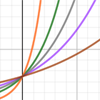# The growth rate of COVID-19

By
Julia Gog, Rachel Thomas, Marianne Freiberger

## The growth rate of COVID-19

### Why isn't R enough?

See here for all our coverage of the COVID-19 pandemic.

We have all become used to thinking about R, the reproduction ratio (or sometimes called the reproduction number) of a disease. That's the number of people infected, on average, by a single infected person. As we saw in previous articles, R helps us understand what is happening with the disease: R>1 means that the epidemic will grow, R=1 means we are plateauing, R<1 means that the epidemic will decline.

(Note that the R we hear about on the news each day changes over the course of the epidemic, it is the effective reproduction number rather than the basic reproduction number, R0, that you might also have heard of – you can find out more about the difference between them here.)

One thing that R does not tell us, though, is how quickly things are changing. This is because R is not a rate, there is no timescale involved. For example, if R=2 for some disease then we know the epidemic will grow (because R>1), but we cannot tell how quickly. For diseases like HIV or TB, where there can be months or years between one person infecting the next person, even R=2 means slow growth over time. However for influenza or measles, where the infection is much faster, on the scale of days, R=2 means very rapid growth.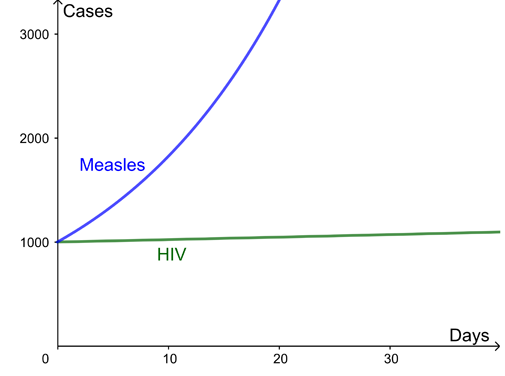Here are two example curves for the growth of infection, both with a reproduction ratio R=2. The difference is the time between new infections – several months for HIV but just days for measles.

### What is the growth rate of an epidemic?

The growth rate of a disease is a natural way to capture how quickly the number of infections are changing day by day. The growth of cases of a disease is modelled using an exponential curve: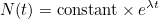Hereis the number of cases, which depends on timemeasured in days, and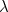(pronounced "lambda") is what is called the growth rate of the disease per day. (The numberis a mathematical constant approximately equal to 2.719 and intimately connected to exponential growth.)

For the example curves above, the growth rate for HIV is=0.002 per day and for measles is=0.06 per day. This leads to wildly different outcomes over the next month despite both diseases in this example having the same reproduction ratio. You can use the interactivity below to explore how the progression of a diseases changes for different growth rates (use the slider to change the value of).

During the COVID-19 pandemic the number of new cases, and new deaths, is reported each day – we watched in horror as these increased in the UK in March and early April, and have now seen them decrease over recent weeks. If the growth rate is positive, the number of new cases each day is increasing, if the growth rate is 0, the number of new cases stays constant. What is needed to keep the epidemic under control is for the growth rate to be negative and hence the number of new cases to be decreasing. If the number of new cases has decreased by 3% since yesterday, then the growth rate is, approximately,= -0.03 per day. (This isn't exactly equivalent but a good approximation for typical values of. The growth rate actually works like compound interest which you can read about here.)

### Which is better: R or the growth rate?

Both the reproduction ratio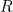, and the growth rate,, are valid measures for understanding the growth of a disease. They each have their uses, as outlined below:

Reproduction ratio: RGrowth rate per day:R is more natural for understanding strength of intervention needed to stop an epidemic, better for planning control measures. For example:

• R=1.5 means we need to reduce transmission by one third.
R thus gives an intuitive way of predicting the strength of future interventions needed to stop an epidemic.

Growth rate is more natural for thinking about how cases change over time. For example

•= 0.01 per day means cases will increase by about 1% a day.
•= -0.02 per day means cases will decrease by about 2% a day.
The growth rate is a good description of what's happening now: if we have a certain number of cases today then we can work out how many to expect tomorrow, the day after, and so on.

R>1 exponential growth
R=1 flat
R<1 exponential decay>0 exponential growth= 0 flat<0 exponential decay

R a ratio of cases by infection generation. It is not a rate: there is no timescale involved. The growth rateis a rate, usually given in days for COVID-19.
R is not at all easy to measure in practice, but can be fitted using models if the timescales of infection are known. In principle it could be estimated by detailed epidemiological data on exactly who got infection from whom, but this is not usually feasible in typical settings. The growth rateis relatively easy to estimate from time series data of cases or deaths (but see below about small numbers). A simple approach is just to find the gradient of the logged cases. More advanced approaches, which can take into account a time-varying growth rate, or heterogeneous population, again involve fitting epidemic models.

Both the reproduction ratio and the growth rate are particularly hard to estimate when the number of cases is small, for example if the incidence of the disease is very low, or if the community you are studying has a very small population. In that case, day to day fluctuations can easily swamp the underlying patterns of the disease, so you will have greater uncertainty about the growth rate (and so expect wider confidence intervals).

### How do you get from R to the growth rate and vice versa?

The precise relationship between R and the growth rate is not straightforward: it needs to take into account the timings of each infection to the next. A crude approximation is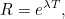where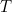is the mean generation time: the time from one infection to the next.

### I can cope with some advanced maths, tell me more!

OK! All of this supposes that the control measures and number of people susceptible to the disease are not changing too quickly.

Following one infected person, denote their time since infection by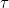(in days). They go on to infectothers on average. For each of these, their timing of infection is distributed with probability density function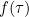. Then (exercise for maths undergraduates!)andare related as follows: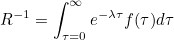and yes, this is very closely related to a Laplace transform, or a moment generating function for the generation time distribution.

For specific distributions for the generation time, for example a gamma distribution, this can sometimes be simplified. Taking the generation time to be exactly constant, say, we recover the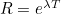, but this is a rather crude approximation for many infectious diseases in practice.

Note,depends on a variety of things, including biological things like the incubation period, and on social factors like whether you still mix with others when you have symptoms or if you self-isolate.

See this paper by Wallinga and Lipsitch for more details.